### Home > CALC > Chapter Ch5 > Lesson 5.4.1 > Problem5-143

5-143.
1. Easy Limits

2. Evaluate each of the following limits. Homework Help ✎

1.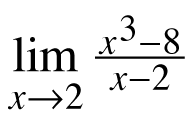2.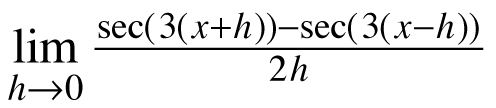3.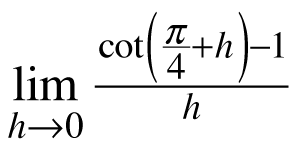4.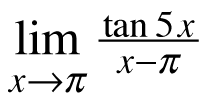5. What do all of the limits above have in common?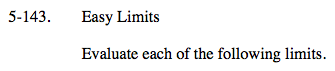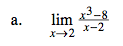You could evaluate this limit algebraically.
Recall that: x3 − 8 = (x − 2)(x2 + 2x − 4)
Or you could recognize that this is Ana's Definition of the Derivative.
f(x) = x3
a = 2
So the limit = f '(2)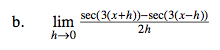You should recognize that this is Hanah's Definition of the Derivative.

Deconstruct Hanah's definition.
1. Determine what is f(x).
2. Find its derivative. That is the value of the limit.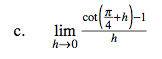This is Hana's Definition of the Derivative, evaluated at

$x=\frac{\pi}{4}.$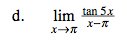This is Ana's Definition of the Derivative, evaluated at
x = π.

This limit could be rewritten as

$\lim_{x\rightarrow \pi}\frac{\text{tan}(5x)-\text{tan}(5\pi)}{x-\pi}$

Of course, tan(5,π) = 0.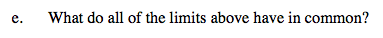Refer to hints above.